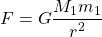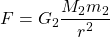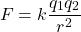# Coulomb’s Law is Gravitic

## Gravity

The particle model describes Newtonian Gravity with a gravitic field (see movement classifications below) of G1 particles. Masses M1 and m1 are pushed together by G1 particles traveling in random directions that hit and pass-through masses M1 and m1.## Strong Force

The Particle Model describes the strong force between charged particles as a second gravitic field of much smaller and faster particles called G2 particles. Masses M2 and m2 (which would be the “charged particles” in mainstream science) are pushed together by G2 particles traveling in random directions that hit and pass-through masses M2 and m2. This is why the Particle Model does not need charge: attraction is caused by pushes from G2 gravitic field.## Similarity Explained

The particle model gives an explanation as to why Coulomb’s Law looks so much like Newton’s gravity quation: both are caused by gravitic fields. They just happen to be different particles at different levels that behave in the same way. Notice how Coulomb’s equation looks very similar to the Particle Model’s equation for attraction of what mainstream science calls “charged particles”:## Movement Classifications

3,682 total views,  1 views today